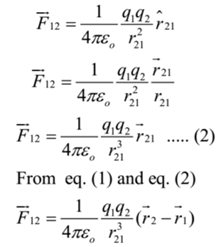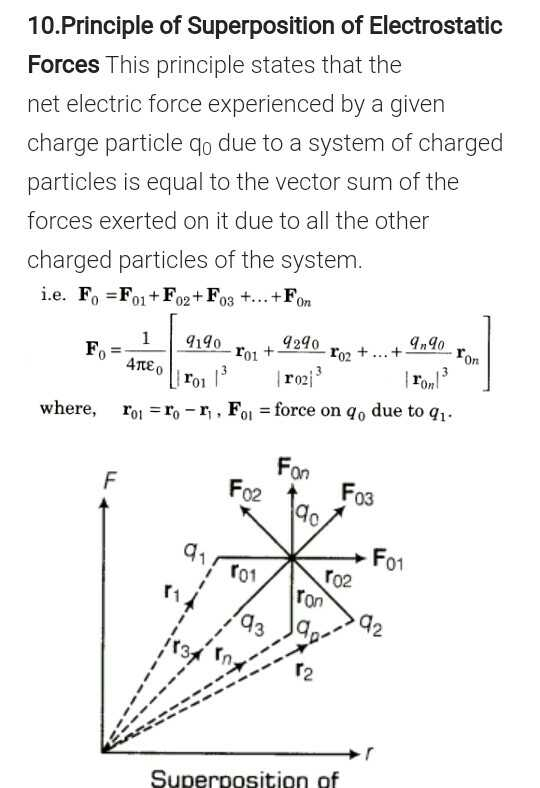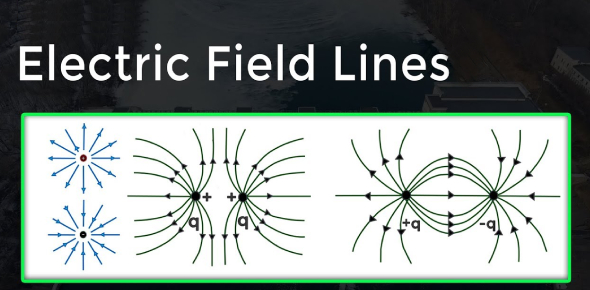# CBSE Class 12 Physics Chapter 1 Revision Notes

Chapter 1: Electric Charges and Fields Revision Notes

• Electric charge: The property of matter that causes it to produce and experience electric and magnetic effects.
• Conductors are substances that readily allow the passage of electricity through them, such as metals and the earth, while insulators are substances that offer high resistance to the passage of electricity, such as plastic rod and nylon.
• Frictional electricity is caused by the transference of electrons.
• Charge Additivity: charges are scalars that add up in the same way that real numbers do. It means that if a system has n charges (q1, q2, q3,…,qn), the total charge will be q1 +q2 +… +qn.
• Charge Conservation: An isolated system’s total charge is always conserved, meaning the system’s initial and final charges are the same.
• Charge Quantification: Charge is quantized because it is a discrete amount rather than a continuous value.
• Charge on an object is calculated as q=+ne, where n is an integer and e is the electronic charge. A physical quantity is said to be quantised when it exists in discrete packets rather than in a continuous amount. As a result, charge is quantified.

## Units of Charge

(i) SI unit coulomb (C)

(ii) CGS system

(a) electrostatic unit, esu of charge or stat-coulomb (stat-C)

(b) electromagnetic unit, emu of charge or ab-C (ab-coulomb)

1 ab-C = 10 C, 1 C = 3 x 109 stat-C

## Coulomb’s law

• It states that electrostatic force of interaction or repulsion acting between two stationary point charges is given bySource

• Coulombian or electrostatic forces) are conservative forces.

## The Principle of Superposition of Electrostatic Forces

• The net electric force experienced by a given charge particle q0 as a result of a system of charged particles is equal to the vector sum of the forces exerted on it by all the other charged particles in the system, according to this principle.Source

## Intensity of the electric field

• The force experienced per unit positive test charge placed at that point without disturbing the source charge is defined as the electric field intensity at any point due to source charge.

## Electric Field Lines

• Electric field lines are a visual representation of the electric field around a charge configuration (s). These lines have a positive charge at the beginning and a negative charge at the end. The direction of the field at any point is determined by the tangent on these lines.
• Positive and negative charge electric field lines, as well as their combinations, are depicted belowSource

### Electric Dipole

• An electric dipole is made up of two point charges of equal magnitude and opposite nature separated by a small distance.
• Dipole Moment: The electric dipole moment (p), which is the product of the charge (q) and the separation between the charges, is used to determine the strength of an electric dipole.
• In a uniform electric field, a dipole is in stable equilibrium when the angle between p and E is 0° and in unstable equilibrium when the angle is 180°.
• In a uniform electric field, the net force on an electric dipole is zero.
• When an electric dipole is placed in a non-uniform electric field, it produces a net force and torque.
• W = pE (cos1 – cos2) is the work done in rotating the electric dipole from 1 to 2.
]]>